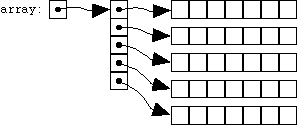## 23.2: Dynamically Allocating Multidimensional Arrays

We've seen that it's straightforward to call malloc to allocate a block of memory which can simulate an array, but with a size which we get to pick at run-time. Can we do the same sort of thing to simulate multidimensional arrays? We can, but we'll end up using pointers to pointers.

If we don't know how many columns the array will have, we'll clearly allocate memory for each row (as many columns wide as we like) by calling malloc, and each row will therefore be represented by a pointer. How will we keep track of those pointers? There are, after all, many of them, one for each row. So we want to simulate an array of pointers, but we don't know how many rows there will be, either, so we'll have to simulate that array (of pointers) with another pointer, and this will be a pointer to a pointer.

This is best illustrated with an example:

```	#include <stdlib.h>

int **array;
array = malloc(nrows * sizeof(int *));
if(array == NULL)
{
fprintf(stderr, "out of memory\n");
exit or return
}
for(i = 0; i < nrows; i++)
{
array[i] = malloc(ncolumns * sizeof(int));
if(array[i] == NULL)
{
fprintf(stderr, "out of memory\n");
exit or return
}
}
```
array is a pointer-to-pointer-to-int: at the first level, it points to a block of pointers, one for each row. That first-level pointer is the first one we allocate; it has nrows elements, with each element big enough to hold a pointer-to-int, or int *. If we successfully allocate it, we then fill in the pointers (all nrows of them) with a pointer (also obtained from malloc) to ncolumns number of ints, the storage for that row of the array. If this isn't quite making sense, a picture should make everything clear:Once we've done this, we can (just as for the one-dimensional case) use array-like syntax to access our simulated multidimensional array. If we write

```	array[i][j]
```
we're asking for the i'th pointer pointed to by array, and then for the j'th int pointed to by that inner pointer. (This is a pretty nice result: although some completely different machinery, involving two levels of pointer dereferencing, is going on behind the scenes, the simulated, dynamically-allocated two-dimensional ``array'' can still be accessed just as if it were an array of arrays, i.e. with the same pair of bracketed subscripts.)

If a program uses simulated, dynamically allocated multidimensional arrays, it becomes possible to write ``heterogeneous'' functions which don't have to know (at compile time) how big the ``arrays'' are. In other words, one function can operate on ``arrays'' of various sizes and shapes. The function will look something like

```	func2(int **array, int nrows, int ncolumns)
{
}
```
This function does accept a pointer-to-pointer-to-int, on the assumption that we'll only be calling it with simulated, dynamically allocated multidimensional arrays. (We must not call this function on arrays like the ``true'' multidimensional array a2 of the previous sections). The function also accepts the dimensions of the arrays as parameters, so that it will know how many ``rows'' and ``columns'' there are, so that it can iterate over them correctly. Here is a function which zeros out a pointer-to-pointer, two-dimensional ``array'':
```	void zeroit(int **array, int nrows, int ncolumns)
{
int i, j;
for(i = 0; i < nrows; i++)
{
for(j = 0; j < ncolumns; j++)
array[i][j] = 0;
}
}
```

Finally, when it comes time to free one of these dynamically allocated multidimensional ``arrays,'' we must remember to free each of the chunks of memory that we've allocated. (Just freeing the top-level pointer, array, wouldn't cut it; if we did, all the second-level pointers would be lost but not freed, and would waste memory.) Here's what the code might look like:

```	for(i = 0; i < nrows; i++)
free(array[i]);
free(array);
```

Read sequentially: prev next up top